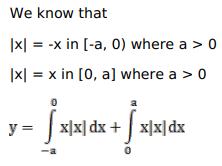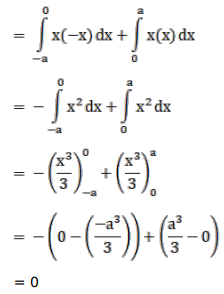# Mark against the correct answer in the following:

Question:

Mark $(\sqrt{)}$ against the correct answer in the following:

$\int_{-a}^{a} x|x| d x=?$

A. 0

B. $2 \mathrm{a}$

C. $\frac{2 a^{3}}{3}$

D. none of these

Solution: